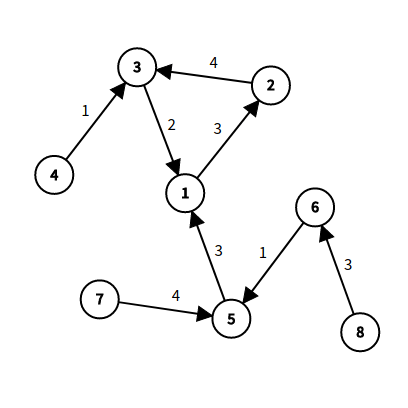# [题解] [IOI2008] Island

$2\le n\le 10^6$

## 思路\begin{aligned} ret1&=\max{f_i+f_j+pre_j-pre_i}\ ret2&=\max{f_i+f_j+len-(pre_j-pre_i)} \end{aligned}

$$ans=\max{ret1,ret2,g_i}$$

• 我们再遍历完一个环之前并没法拿到这个环的长度 $len$，因此我们更新 $ret2$ 时不加上 $len$，最后遍历完后再给 $ret2$ 加上 $len$ 即可。

• 我们可以把 $g_i$ 顺便统计到 $ret1$ 里面，并没有什么用

## 代码

494ms / 30.84MB，目前洛谷2nd最优解

mivik.h

Mivik

2020-08-23

2022-11-11